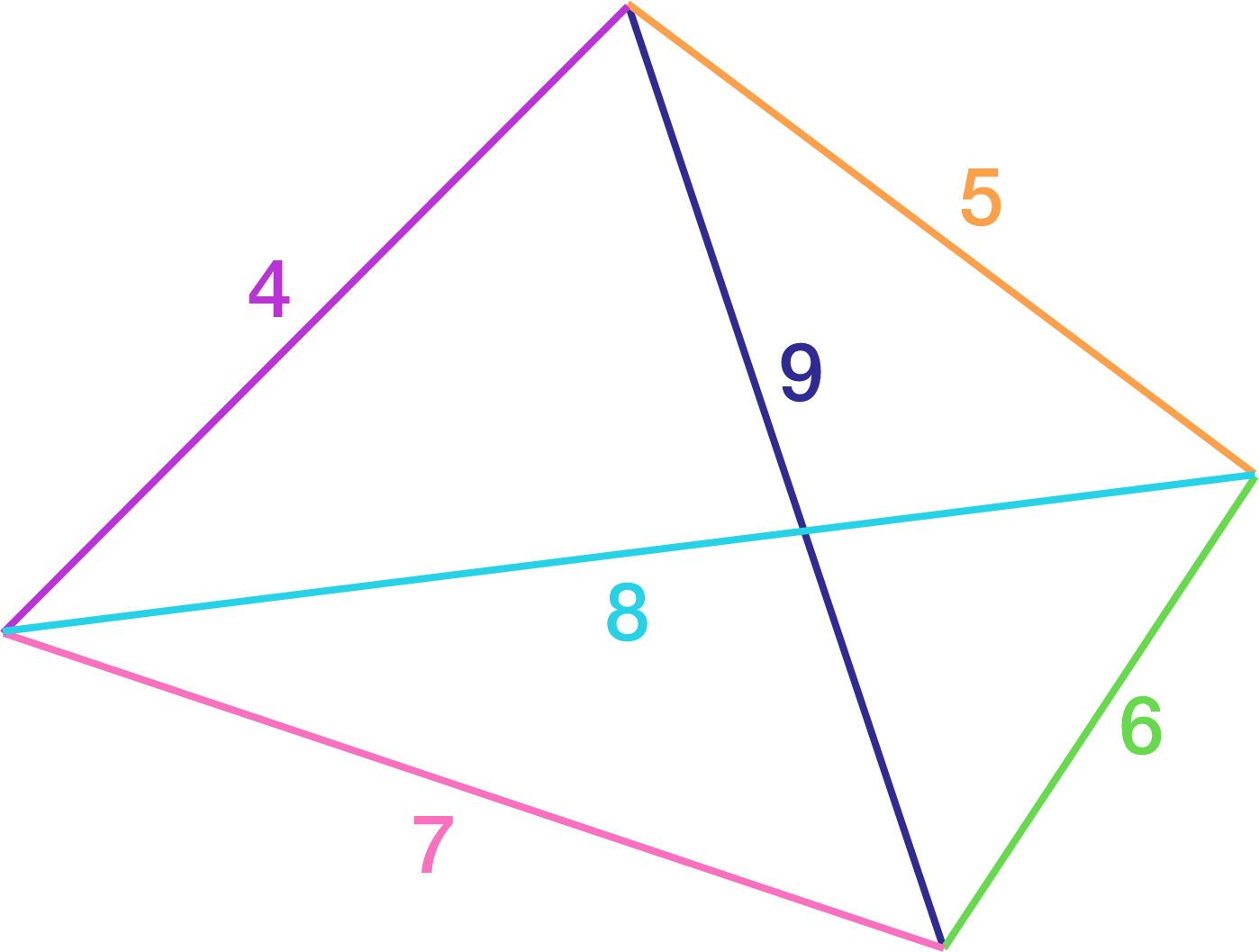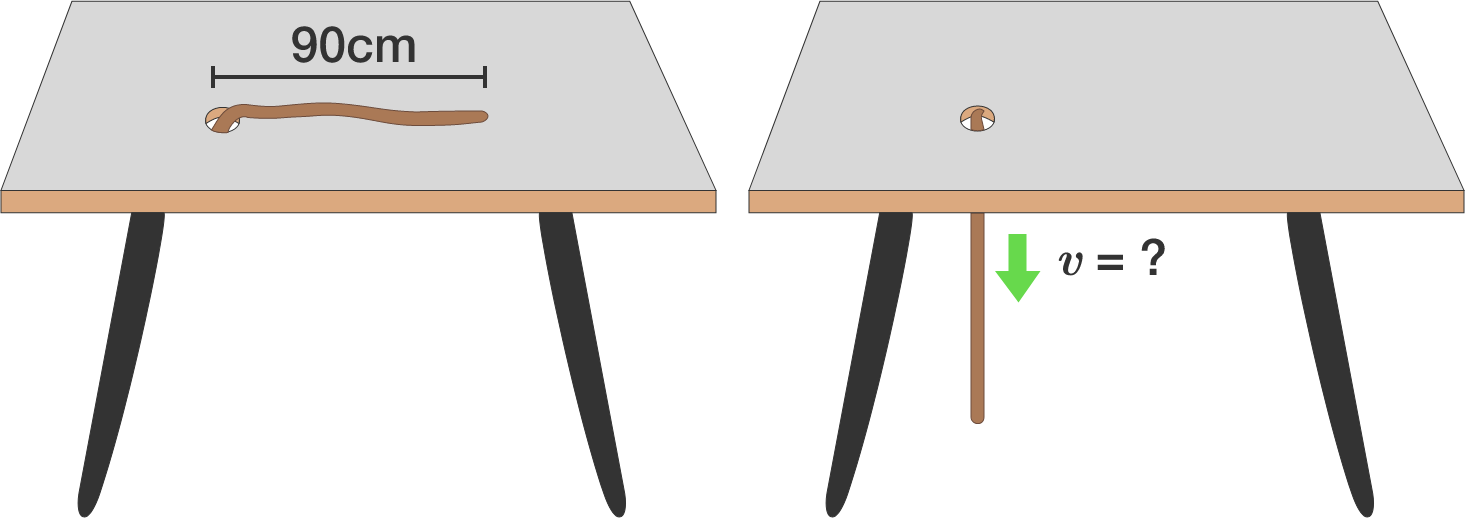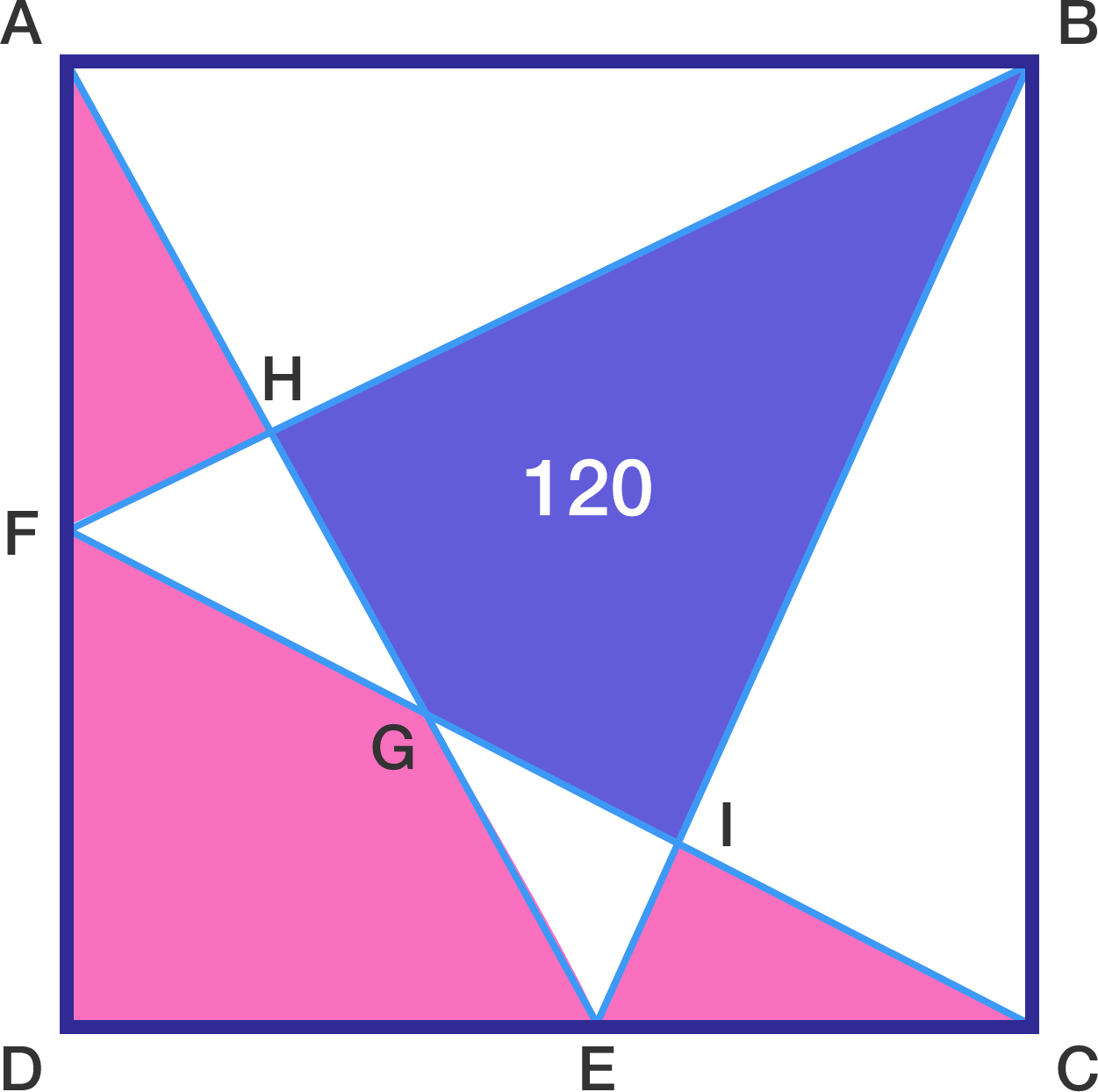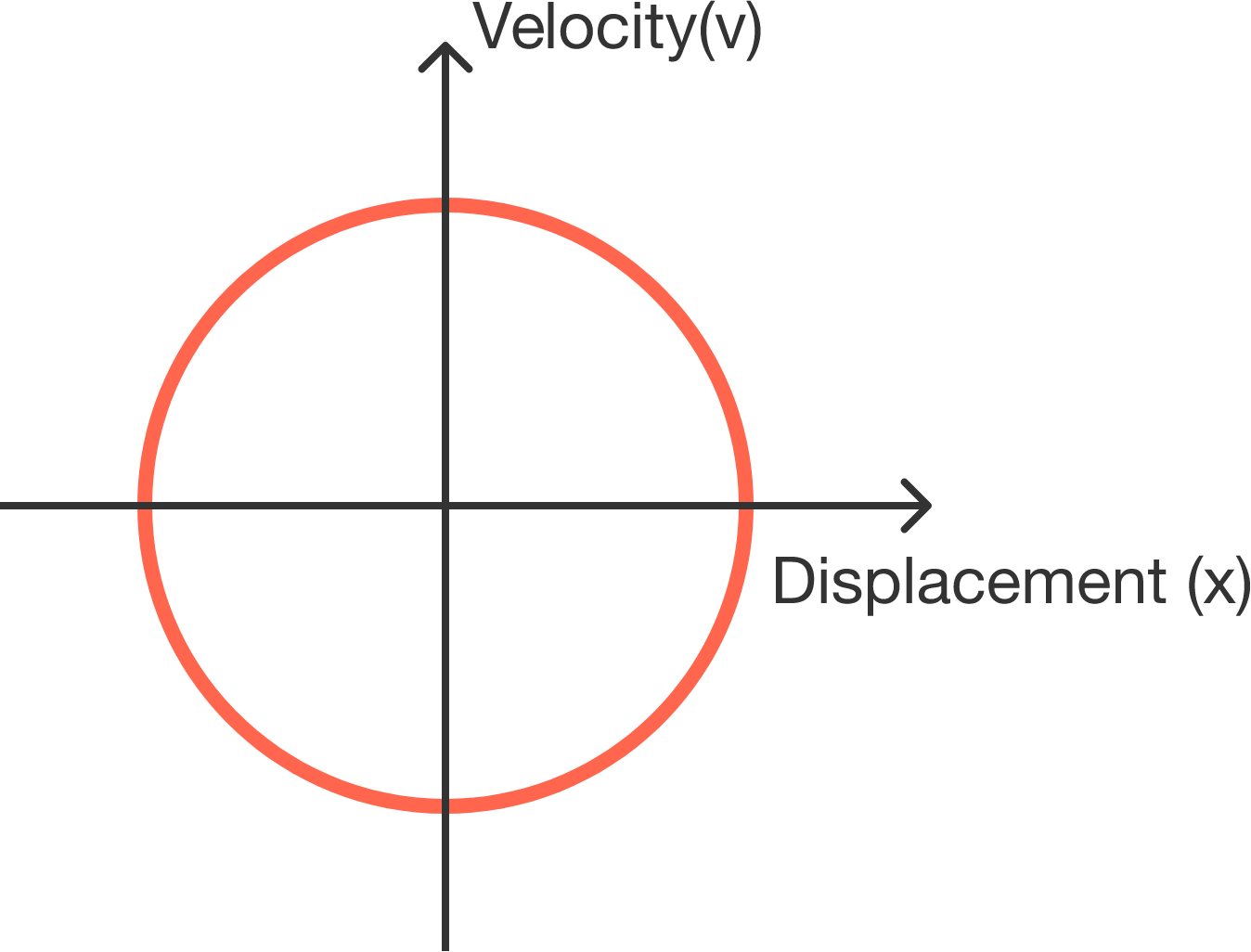# Problems of the Week

Contribute a problem

# 2017-04-17 IntermediateDoes there exist a quadrilateral with the side lengths and diagonal lengths as indicated above?

Note: We are working in $\mathbb{R}^2$ with the usual Euclidean geometry.A rope of length $\SI{90}{\centi\meter}$ lies in a straight line on a frictionless table, except for a very small piece at one end which hangs down through a hole in the table.

This piece is released, and the rope slides down through the hole. What is the speed $($in $\text{m/s})$ of the rope (to 2 decimal places) at the instant it loses contact with the table?

Details: $g = \SI[per-mode=symbol]{9.81}{\meter\per\second\squared}.$$ABCD$ is a square with points $E$ and $F$ lying on sides $CD$ and $AD,$ respectively. If the purple area is $[BHGI]=120,$ what is the sum of the pink areas $[AHF]+[FGED]+[ICE]?$A particle moves in 1-dimension. If we plot its velocity and displacement over time, the trajectory forms a circle that's centered at the origin.

Which of the following relations is true regarding its acceleration $(a),$ velocity $(v),$ and displacement $(x)$?

Note: In the options, $k$ is a positive constant.There are $n$ people in a room, who each wear a hat of a specific color. They are able to see other people's hats but not their own.

One of them shouted, "If you can see at least 5 red hats and at least 5 white hats, raise your hand!"

Exactly 10 people raised their hands.

What is the minimum value of $n$ that fits this scenario?

×